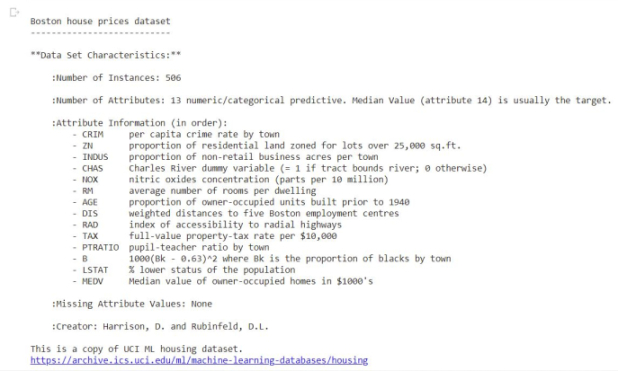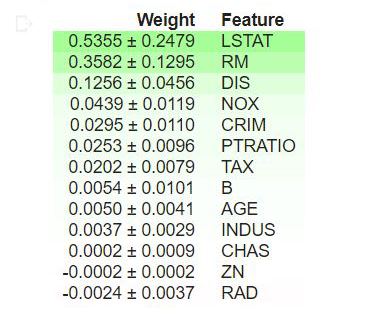Related Articles

# Machine Learning Explainability using Permutation Importance

• Last Updated : 18 Jul, 2021

Machine learning models often act as black boxes, meaning that they can make good predictions but it is difficult to fully comprehend the decisions that drive those predictions. Gaining insights from a model is not an easy task, despite the fact that they can help with debugging, feature engineering, directing future data collection, informing human decision-making, and finally, building trust in a model’s predictions.

One of the most trivial queries regarding a model might be determining which features have the biggest impact on predictions, called feature importance. One way to evaluate this metric is permutation importance

Permutation importance is computed once a model has been trained on the training set. It inquires: If the data points of a single attribute are randomly shuffled (in the validation set), leaving all remaining data as is, what would be the ramifications on accuracy, using this new data?

Ideally, random reordering of a column ought to result in reduced accuracy, since the new data has little or no correlation with real-world statistics. Model accuracy suffers most when an important feature, that the model was quite dependent on, is shuffled. With this insight, the process is as follows:

1. Get a trained model.
2. Shuffle the values for a single attribute and use this data to get new predictions. Next, evaluate change in loss function using these new values and predictions, to determine the effect of shuffling. The drop in performance  quantifies the importance of the feature that has been shuffled.
3. Reverse the shuffling done in the previous step to get the original data back.  Redo step 2 using the next attribute, until the importance for every feature is determined.

Python’s ELI5 library provides a convenient way to calculate Permutation Importance. It works in Python 2.7 and Python 3.4+. Currently it requires scikit-learn 0.18+. You can install ELI5 using pip:

`pip install eli5`

or using:

`conda install -c conda-forge eli5`

We’ll train a Random Forest Regressor using scikitlearn’s Boston Housing Prices dataset, and use that trained model to calculate Permutation Importance.

## Python3

 `from` `sklearn.datasets ``import` `load_boston`` ` `boston ``=` `load_boston()``print``(boston.DESCR[``20``:``1420``])`

Output:## Python3

 `from` `sklearn.model_selection ``import` `train_test_split`` ` `# separate data into target & independent variables``x ``=` `boston.data``y ``=` `boston.target`` ` `# split data into train and test set``x_train, x_test, y_train, y_test ``=` `train_test_split(x, y, train_size``=``0.8``)``print``(``'Size of: '``)``print``(``'Training Set x: '``, x_train.shape)``print``(``'Training Set y: '``, y_train.shape)``print``(``'Test Set x: '``, x_test.shape)``print``(``'Test Set y: '``, y_test.shape)`

Output:

```Size of:
Training Set x: (404, 13)
Training Set y: (404,)
Test Set x: (102, 13)
Test Set y: (102,)
```

## Python3

 `from` `sklearn.ensemble ``import` `RandomForestRegressor`` ` `# train model on training set``rf ``=` `RandomForestRegressor()`` ` `# fit model on training set``rf.fit(x_train, y_train)`` ` `# calculate score on test set``print``(``'R2 score for test set: '``)``print``(rf.score(x_test, y_test))`

Output:

```R2 score for test set: 0.857883705095584
```

## Python3

 `import` `eli5``from` `eli5.sklearn ``import` `PermutationImportance`` ` `# create permutation importance object using model``# and fit on test set``perm ``=` `PermutationImportance(rf, random_state``=``1``).fit(x_test, y_test)`` ` `# display weights using PermutationImportance object``eli5.show_weights(perm, feature_names ``=` `boston.feature_names)`

Output:## Interpretation

• The values at the top of the table are the most important features in our model, while those at the bottom matter least.
• The first number in each row indicates how much model performance decreased with random shuffling, using the same performance metric as the model (in this case, R2 score).
• The number after the ± measures how performance varied from one-reshuffling to the next, i.e., degree of randomness across multiple shuffles.
• Negative values for permutation importance indicate that the predictions on the shuffled (or noisy) data are more accurate than the real data. This means that the feature does not contribute much to predictions (importance close to 0), but random chance caused the predictions on shuffled data to be more accurate. This is more common with small datasets.

In our example, the top 3 features are LSTAT, RM and DIS, while the 3 least significant are RAD, CHAS and ZN.

## Summary

This article is a brief introduction to Machine Learning Explainability using Permutation Importance in Python. Gaining intuition into the impact of features on a model’s performance can help with debugging and provide insights into the dataset, making it a useful tool for data scientists.

## References

Attention reader! Don’t stop learning now. Get hold of all the important Machine Learning Concepts with the Machine Learning Foundation Course at a student-friendly price and become industry ready.

My Personal Notes arrow_drop_up